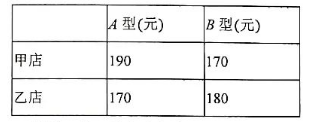(1) 设分配给甲电商专卖店 $A$ 型产品 $x$ 件 $(20 \leq x \leq 80$ ), 如果记这家服装公司菄出这 200 件童装的总利润为 $y$ (元), 求 $y$ 关于 $x$ 的函数关系式:
(2)如果要使得总利润最大，服装厂应当如何分配？最大利润是多少?
【答案】 （1）$\quad y=(190-90) x+(170-80)(140-x)+(170-90)(80-x)+(180-80)(x-20)$
$y=30 x+17000 \quad(20 \leq x \leq 80)$

（2）由 $y=30 x+17000 \quad(20 \leq x \leq 80)$
$\therefore 30 > 0$ y随x的增大而增大
$x=80$ 时， $y_{\text {max }}=30 \times 80+17000=194000$